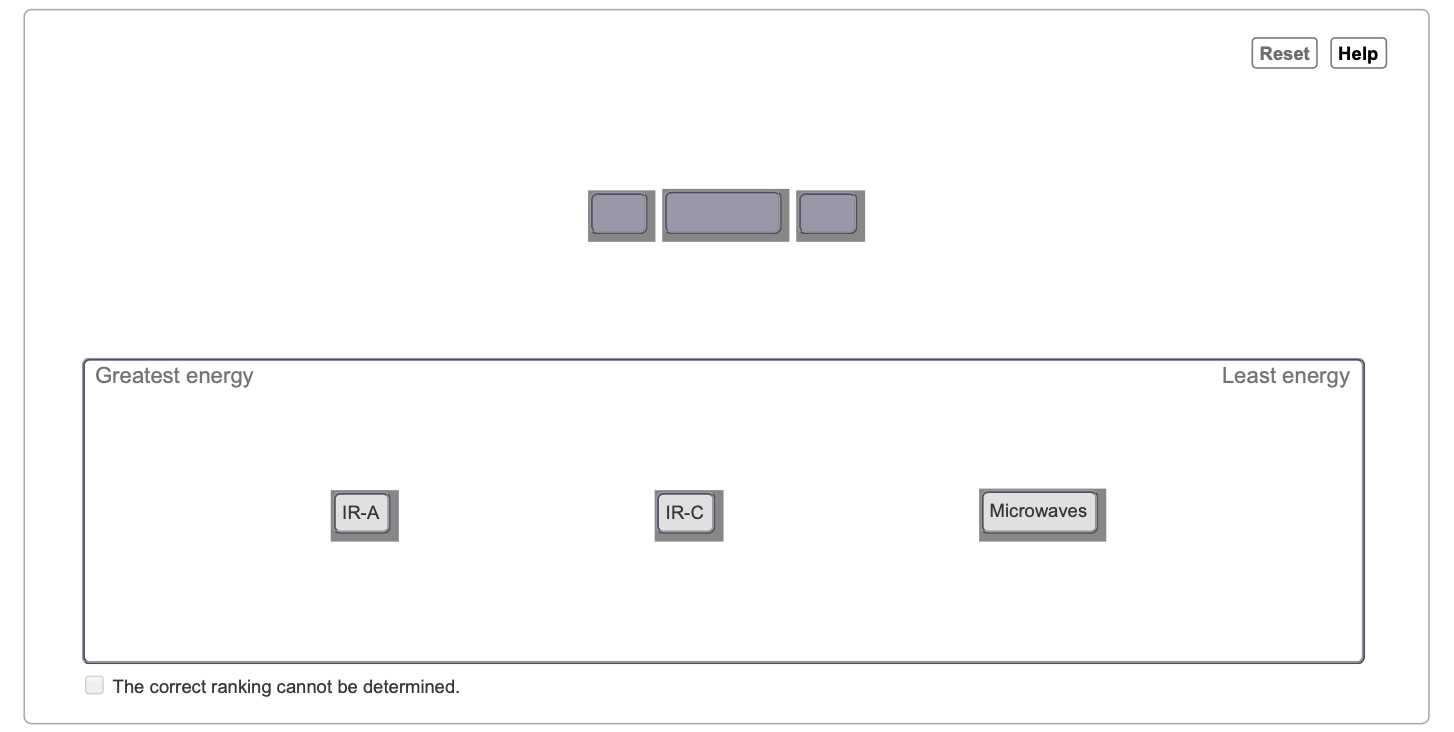# Problem: Part B. Two of the types of infrared light, IR-C and IR-A, are both components of sunlight. Their wavelengths range from 3000 to 1,000,000 nm for IR-C and from 700 to 1400 nm for IR-A. Compare the energy of microwaves, IR-C, and IR-A.Rank from greatest to least energy per photon. To rank items as equivalent, overlap them.Electromagnetic radiation behaves both as particles (called photons) and as waves. Wavelength (λ) and frequency (ν) are related according to the equation:c=λ×νwhere c is the speed of light (3.00 × 108 m/s). The energy (E in joules) contained in one quantum of electromagnetic radiation is described by the equation:E=h×νwhere h is Planck's constant (6.626 × 10−34 J•s). Note that frequency has units of inverse seconds (s−1), which are more commonly expressed as hertz (Hz).

###### FREE Expert Solution
100% (71 ratings)View Complete Written Solution
###### Problem Details

Part B. Two of the types of infrared light, IR-C and IR-A, are both components of sunlight. Their wavelengths range from 3000 to 1,000,000 nm for IR-C and from 700 to 1400 nm for IR-A. Compare the energy of microwaves, IR-C, and IR-A.Rank from greatest to least energy per photon. To rank items as equivalent, overlap them.

Electromagnetic radiation behaves both as particles (called photons) and as waves. Wavelength (λ) and frequency (ν) are related according to the equation:

c=λ×ν

where c is the speed of light (3.00 × 108 m/s). The energy (E in joules) contained in one quantum of electromagnetic radiation is described by the equation:

E=h×ν

where h is Planck's constant (6.626 × 10−34 J•s). Note that frequency has units of inverse seconds (s−1), which are more commonly expressed as hertz (Hz).

Frequently Asked Questions

What scientific concept do you need to know in order to solve this problem?

Our tutors have indicated that to solve this problem you will need to apply the Electromagnetic Spectrum concept. You can view video lessons to learn Electromagnetic Spectrum. Or if you need more Electromagnetic Spectrum practice, you can also practice Electromagnetic Spectrum practice problems.

What professor is this problem relevant for?

Based on our data, we think this problem is relevant for Professor Selegue's class at PIMA.